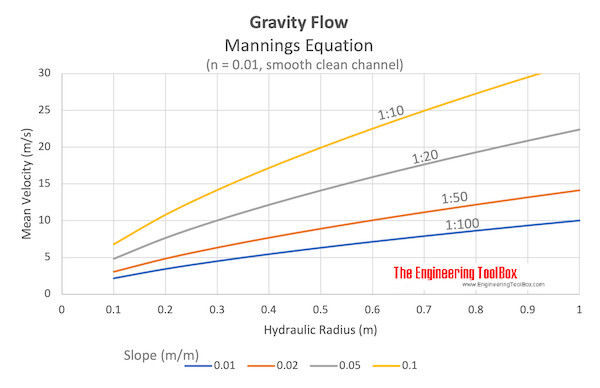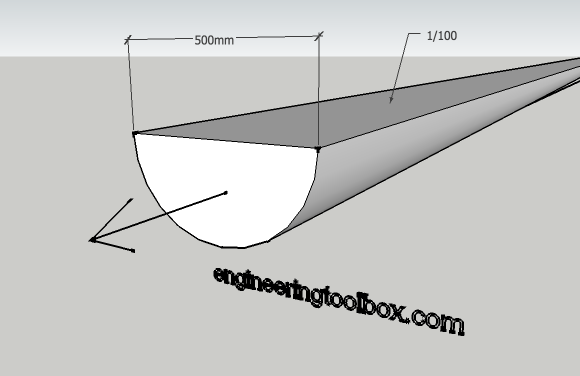Engineering ToolBox - Resources, Tools and Basic Information for Engineering and Design of Technical Applications!

# Manning's Formula and Gravity Flow

## Calculate cross-sectional average velocity flow in open channels.

Manning's equation can be used to calculate cross-sectional average velocity flow in open channels

v = (kn / n) Rh2/3 S1/2                       (1)

where

v = cross-sectional mean velocity (ft/s, m/s)

kn = 1.486 for English units and kn = 1.0 for SI units

n = Manning coefficient of roughness - ranging from 0.01 (a clean and smooth channel) to 0.06 (a channel with stones and debris, 1/3 of vegetation)

Rh = hydraulic radius (ft, m)

S = slope - or gradient - of pipe (ft/ft, m/m)

Hydraulic radius can be expressed as

Rh = A / Pw                             (2)

where

A = cross sectional area of flow (ft2, m)

Pw = wetted perimeter (ft, m)The volume flow in the channel can be calculated as

q = A v = A (kn / n) Rh2/3 S1/2                         (3)

where

q = volume flow (ft3/s, m3/s)

A = cross-sectional area of flow (ft2, m2)

### Example - Flow in an Open Channel

A channel with the shape of an half circle is 100% filled. The diameter of the half circle is 500 mm (0.5 m) and the channel is made of concrete with Manning coefficient 0.012. The slope of the channel is 1/100 m/m.make 3D models with the free Engineering ToolBox Sketchup Extension!

The cross section area of the half circle flow can be calculated as

A = (0.5 π ((0.5 m) / 2)2)

= 0.098 m2

The wetted perimeter of the half circle flow can be calculated as

P = 0.5 2 π (0.5 m) / 2)

= 0.785 m

The hydraulic radius of the channel can be calculated from (2) as

Rh = A / P

= (0.098 m2) / (0.785 m)

= 0.125 m

The cross sectional mean velocity can be calculated from (1) as

v = (kn / n) Rh2/3 S1/2

= (1.0 / 0.012) (0.125 m)2/3 (1/100 m/m)1/2

= 2.1 m/s

The volume flow can be calculated from (3) as

q = A v

=  (0.098 m2) (2.1 m/s)

= 0.20 m3/s

### Gravity Flow Calculator - Half Filled Circular Pipe

The Gravity Flow Calculator is based on the equations and the example above above. It's valid for half filled circular pipe.

Diameter of pipe (m, ft)

Slope (m/m, ft/ft)

n (1.0 for SI units, 1.486 for Imperial units)

Manning coefficient of roughness

## Related Topics

• Fluid Mechanics - The study of fluids - liquids and gases. Involving velocity, pressure, density and temperature as functions of space and time.
• Flow Measurement - Flow metering principles - Orifice, Venturi, Flow Nozzles, Pitot Tubes, Target, Variable Area, Positive Displacement, Turbine, Vortex, Electromagnetic, Ultrasonic Doppler, Ultrasonic Time-of-travel, Mass Coriolis, Mass Thermal, Weir V-notch, Flume Parshall and Sluice Gate flow meters and more.

## Engineering ToolBox - SketchUp Extension - Online 3D modeling!

Add standard and customized parametric components - like flange beams, lumbers, piping, stairs and more - to your Sketchup model with the Engineering ToolBox - SketchUp Extension - enabled for use with the amazing, fun and free SketchUp Make and SketchUp Pro .Add the Engineering ToolBox extension to your SketchUp from the SketchUp Pro Sketchup Extension Warehouse!

Translate

## Privacy

We don't collect information from our users. Only emails and answers are saved in our archive. Cookies are only used in the browser to improve user experience.

Some of our calculators and applications let you save application data to your local computer. These applications will - due to browser restrictions - send data between your browser and our server. We don't save this data.

## Citation

• Engineering ToolBox, (2004). Manning's Formula and Gravity Flow. [online] Available at: https://www.engineeringtoolbox.com/mannings-formula-gravity-flow-d_800.html [Accessed Day Mo. Year].

Modify access date.

. .

#### Scientific Online Calculator3 10